# why direction of dipole movement is from negative topositive charge.?Saurabh Kumar
8 years ago
There is no particular way of describing the direction of dipole rather it is hypothetic.But one should know the fact that if lenth is taken as 2L,means it has a specific origion(No matter for ease it is taken).Now as possible,draw a graph of dipole and its axial line co-insided with the X-axis.Therefore we will find the -ve charge takes the position on left of the origion shows charge is also negative.Now leave all the quadrants except when all trig.functions are +ve..i.e Quad.1st....Draw a point on axis on this quadrant,now predict the direction,we say it is from centre 'O' towards that point.i.e we are supposing its direction from origion to positive....i.e from left to right if direction is obtained from the pont left of origion to the point under consideration,we have to follow same rules.Same is in dipole direction i.e from -ve to +ve......Other all stories are hypothetical...
8 years ago
This may be explained as below.  Look at the diagram below: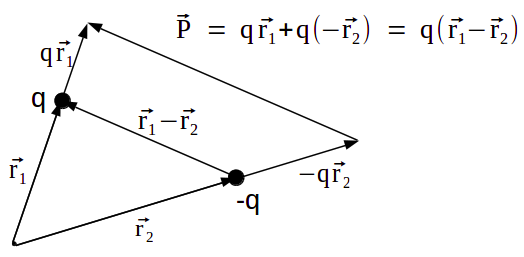p { margin-bottom: 0.25cm; line-height: 120%; }

Charges q and –q are at position vectors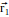and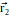respectively from an arbitrary origin. Moment of the charge q about chosen origin is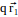and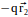respectively. Now dipole moment of the system is equal to vector sum of moments of q and –q, which is given by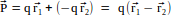. Now we can see that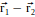is pointing from –q to +q. Hence dipole moment is vec P is pointing from –q to +q.

8 years ago
p { margin-bottom: 0.25cm; line-height: 120%; }

Ooops... there is a small mistake in the diagram. The vectorshowing the moment of charge –q should point in opposite direction.